Electron. J. Diff. Equ., Vol. 2015 (2015), No. 85, pp. 1-18.

### Ground states for a modified capillary surface equation in weighted Orlicz-Sobolev space Guoqing Zhang, Huiling Fu

Abstract:
In this article, we prove a compact embedding theorem for the weighted Orlicz-Sobolev space of radially symmetric functions. Using the embedding theorem and critical points theory, we prove the existence of multiple radial solutions and radial ground states for the following modified capillary surface equation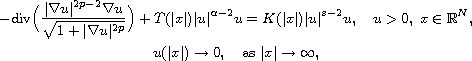where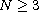,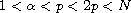,satisfies some suitable conditions,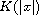and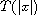are continuous, nonnegative functions.

Submitted August 6, 2014. Published March 7, 2015.
Math Subject Classifications: 35J65, 35J70.
Key Words: Compact theorem; modified capillary surface equation; weighted Orlicz-Sobolev space; ground state.

Show me the PDF file (297 KB), TEX file, and other files for this article.Guoqing Zhang College of Sciences University of Shanghai for Science and Technology Shanghai 200093, China email: shzhangguoqing@126.com Huiling Fu College of Sciences University of Shanghai for Science and Technology Shanghai 200093, China email: fuhuiliing80@163.com